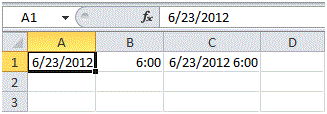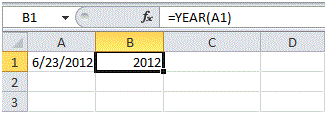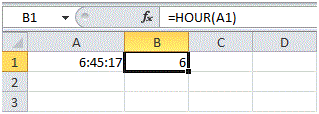# Date and Time Function in Excel

## Date and Time Function in Excel

MS Excel supports multiple formats for data inputs. It also support date and time as a format and has the provision to apply various function over them.

Date Function

Entering date in MS Excel is a little bit different from entering any text or number value. Since the date value has 3 parts therefore, you will need to use separators. Here, MS Excel supports 2 types of separators for Date. You may either use “/” or “-“characters.Note: The date shown in figure above, is in US Format where Months comes first, followed by Days and finally the year. This format depends on windows regional settings.

As it is mentioned earlier that MS Excel supports functions on Dates. Here are few functions that you will learn in this tutorial.

Year, Month, Day

To extract the year part from the date, type =YEAR(“Cell Ref”). This will return the year part as shown in the figure below.Similarly, Month and Day function can be applied in same manner to obtain Month and Day part of the date.

You can add number of days to a date by using the following simple formula as shown in the figure below.Adding Years, Month and Days in Date

You can add number of years, months and days to a date by using the following formula as shown in the figure below.In the above formula, Date function has been used [=Date(Year,Month,Day)] with the addition of 4 in year’s part, 2 in month’s part and 9 in day’s part.

Current Date & Time

If you want to get the current system date and time in MS Excel, you can use NOW() function.If you desire to get the today’s date only then you can use TODAY() function.

Time

Similar to Date, MS Excel also supports Time as a format and various functions can be applied to Time values. To enter time in MS Excel, you can use “:” character as a separator.

Hour, Min, Sec

Similar to Date functions, you can also get the Hour, Minute or Second part of the Time value by using the formula shown in the figure below.Note: You can use the MINUTE and SECOND function to return the minute part and second part of the time.

Time Function

You can add hours, minutes or seconds in the time by using the following formula as shown in the figure below.In the above formula, TIME function has been used [=TIME(Hour, Minute, Second)] with the addition of 2 in hour’s part, 10 in minute’s part and 70 in second’s part.